Download Presentation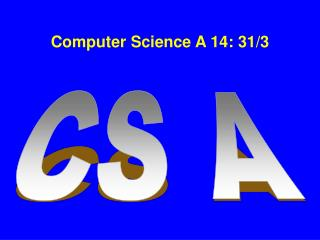Computer Science A 14: 31/3

# Computer Science A 14: 31/3 - PowerPoint PPT Presentation

Computer Science A 14: 31/3. CS A. Inheritence. You can extend a class or change behaviour. Inheritance is a method to reuse code. Concept. Extending classes with fields and methods Late binding Casting objects. Example. class Point{ int x; int y; Point(int x,int y){I am the owner, or an agent authorized to act on behalf of the owner, of the copyrighted work described.
Download Presentation## Computer Science A 14: 31/3

An Image/Link below is provided (as is) to download presentation

Download Policy: Content on the Website is provided to you AS IS for your information and personal use and may not be sold / licensed / shared on other websites without getting consent from its author.While downloading, if for some reason you are not able to download a presentation, the publisher may have deleted the file from their server.

- - - - - - - - - - - - - - - - - - - - - - - - - - E N D - - - - - - - - - - - - - - - - - - - - - - - - - -
Presentation Transcript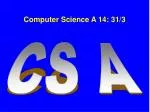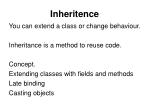Inheritence

You can extend a class or change behaviour.

Inheritance is a method to reuse code.

Concept.

Extending classes with fields and methods

Late binding

Casting objects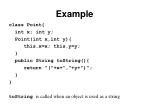Example

class Point{

int x; int y;

Point(int x,int y){

this.x=x; this.y=y;

}

public String toString(){

return "("+x+","+y+")";

}

}

toString is called when an object is used as a string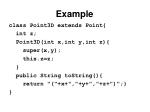Example

class Point3D extends Point{

int z;

Point3D(int x,int y,int z){

super(x,y);

this.z=z;

}

public String toString(){

return "("+x+","+y+","+z+")";}

}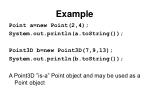Example

Point a=new Point(2,4);

System.out.println(a.toString());

Point3D b=new Point3D(7,9,13);

System.out.println(b.toString());

A Point3D ”is-a” Point object and may be used as a Point object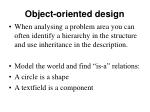Object-oriented design
• When analysing a problem area you can often identify a hierarchy in the structure and use inheritance in the description.
• Model the world and find “is-a” relations:
• A circle is a shape
• A textfield is a component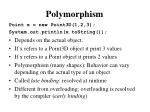Polymorphism

Point x = new Point3D(1,2,3);

System.out.println(x.toString());

• Depends on the actual object.
• If x refers to a Point3D object it print 3 values
• If x refers to a Point object it prints 2 values
• Polymorphism (many shapes): Behavior can vary depending on the actual type of an object
• Called late binding: resolved at runtime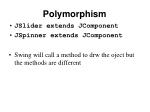Polymorphism
• JSlider extends JComponent
• JSpinner extends JComponent
• Swing will call a method to drw the oject but the methods are different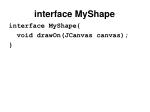interface MyShape

interface MyShape{

void drawOn(JCanvas canvas);

}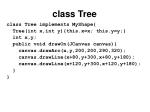class Tree

class Tree implements MyShape{

Tree(int x,int y){this.x=x; this.y=y;}

int x,y;

public void drawOn(JCanvas canvas){

canvas.drawArc(x,y,200,200,290,320);

canvas.drawLine(x+80,y+300,x+80,y+180);

canvas.drawLine(x+120,y+300,x+120,y+180);

}

}class Car

class Car implements MyShape{

Car(int x,int y){this.x=x; this.y=y;}

int x,y;

public void drawOn(JCanvas canvas){

canvas.drawRect(x,y+50,300,50);

canvas.drawLine(x+50,y+50,x+100,y);

canvas.drawLine(x+100,y,x+200,y);

canvas.drawLine(x+200,y,x+250,y+50);

canvas.drawOval(x+50,y+100,50,50);

canvas.drawOval(x+200,y+100,50,50);

}

}class MyShapeTest

port javax.swing.*;

public class MyShapeTest{

public static void main(String args[]){

JFrame frame=new JFrame("MyShapes");

frame.setSize(600,600);

frame.setDefaultCloseOperation(JFrame.EXIT_ON_CLOSE);

JCanvas canvas=new JCanvas();

MyShape s1=new Car(10,300);

MyShape s2=new Car(270,400);

MyShape s3=new Tree(330,40);

s1.drawOn(canvas);

s2.drawOn(canvas);

s3.drawOn(canvas);

frame.setVisible(true);

}

}And more…

An interface is a placeholder for a number of classes.

A class can be an extension of only one class but it may implement several interfaces